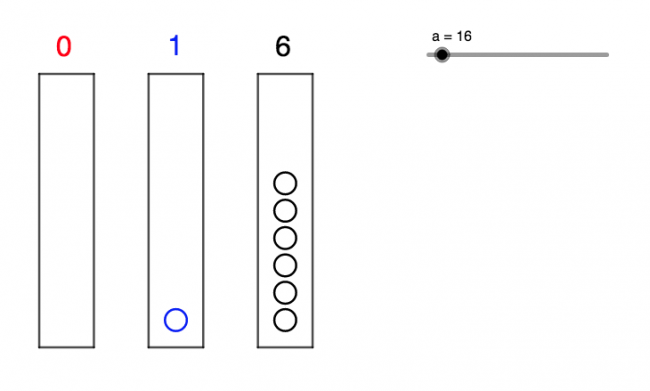# Animation of Place Value

Preview:This Geogebra interactive allows students to see how place value changes as one increases the numbers and visualize place value as the filling of different bins representing different values.

Technology
Curricular Competency:
Reasoning and analyzing: Use technology to explore mathematics
Understanding and solving: Develop, demonstrate, and apply mathematical understanding through play, inquiry, and problem solving
Understanding and solving: Visualize to explore mathematical concepts
Communicating and representing: Communicate mathematical thinking in many ways
Communicating and representing: Represent mathematical ideas in concrete, pictorial, and symbolic forms